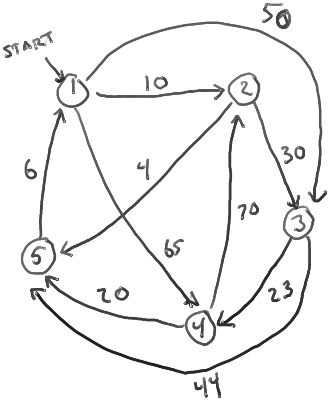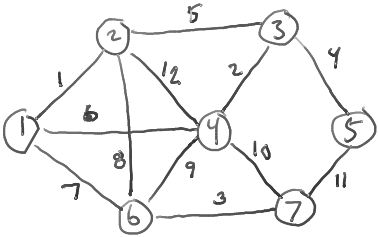# ClassS04CS141/Hwk5Soln

ClassS04CS141 | ClassS04CS141 | recent changes | Preferences

No diff available--this is the first major revision. (no other diffs)

## Hwk5, due Monday, week 9, at start of class

1. Run Dijkstra's algorithm on the following directed graph, starting at vertex 1. After each iteration of the outer loop, show the contents of the queue Q (the vertices in the queue and their keys). What are the final distances (in the distance[] array)?iteration 0: Q={vertex 1 with key 0}
iteration 1: Q={vertex 2 with key 10, vertex 3 with key 50, vertex 4 with key 65} (distance = 0)
iteration 2: Q={vertex 3 with key 40, vertex 4 with key 65, vertex 5 with key 14} (distance = 10)
iteration 3: Q={vertex 3 with key 40, vertex 4 with key 65} (distance = 14)
iteration 4: Q={vertex 4 with key 63} (distance = 40)
iteration 5: Q={} (distance = 63)

2. The bottleneck of a path is the smallest weight WT(u,v) of any edge (u,v) on the path. Given a directed graph G=(V,E) with edge wts and a source vertex s, define, for each vertex v,
max_bottleneck(s,v) = the maximum, over all paths p from s to v, of the bottleneck of p.
The maximum bottleneck paths problem is (given G, s, and WT), to compute max_bottleneck(s,v) for each vertex v.

(For example, suppose the vertices represent cities, the edges represent roads, and the weight WT(u,v) of an edge (u,v) is the clearance of the lowest bridge on the road from u to v. Then max_bottleneck(s,v) is the height of the largest truck that can get from s to v.)

2A: For each vertex v in the graph for problem 1, what is max_bottleneck(s,v)?

Assuming s=1, mb(s,1) = ∞, mb(s,2) = 23, mb(s,3) = 50, mb(s,4) = 65, mb(s,5) = 44

2B: Describe a linear-time algorithm for maximum bottleneck paths that works for acyclic digraphs. Give a high-level description and pseudo-code.

High-level description:

The maximum bottleneck of any path from s to w is the maximum bottleneck of any path from s through any immediate predecessor v of w to w. This gives the following recurrence: mb(s,v) = max { min{WT(w,u), mb(s,w)} : (w,u) ∈ E }. Use this recurrence and dynamic programming to compute the max bottlenecks:

``` 1. Consider the vertices in topological order.
2. Set mb(s,s) = ∞.
3. For each vertex v, set mb(s,v) = max { min{WT(w,u), mb(s,w)} : (w,u) ∈ E }.
```

3. A <i>spanning tree<\i> of a undirected graph G is a tree (a connected, acyclic subgraph) containing all the vertices of G.

If G has edge weights, the <i>weight of a tree T is the sum of the weights of the edges in the tree T.

The minimum spanning tree problem is to find a spanning tree T (of a graph G with edge weights) of minimum possible weight.3A: What is the weight of the minimum-weight spanning tree in the graph above? What are the edges of the tree?

The weight is 1+2+3+4+5+7 = 22.

The edges are the edges labelled 1,2,3,4,5, and 7.

3B: Claim: in any graph G with edge weights, there is only one spanning tree of minimum total weight. (That is, if T and T' are two different spanning trees, then only one of them can be a minimum spanning tree.)

Prove or disprove this claim.

The claim is false. Consider a 3-cycle with edges of weight 1. Then any two edges form an MST.

3C: Claim: in any graph G, the highest-weight edge cannot be in any minimum spanning tree of G.

Prove or disprove this claim.

The claim is false. Consider a graph with two vertices connected by one edge of weight 1.

4. Suppose you have a directed graph G=(V,E) with non-negative vertex weights (that is, every vertex v is assigned a numeric weight WT(v)). You have a start vertex S and a destination vertex T, and you want to find a path from S to T such that the total weight of the vertices along the path is as small as possible.

Describe how you would design an algorithm to do this.

``` 1. Assign weights on the edges as follows:  WT(u,v) = WT(u).
2. Find a shortest path from S to T of minimum length (based on edge weights) using Dijkstra's algorithm.
```

That path will also minimize the total weight of the vertices on any s->t path.

5. Review the algorithm for single-source shortest paths by dynamic programming. (Bellman-Ford algorithm, summarized here.)

Modify this algorithm to instead compute single-source maximum bottleneck paths (defined in problem 2, above.) Describe the high-level idea of your modification, and give pseudo-code too.

We use the same structure -- dynamic programming based on the structure of the paths. However, we change the recurrence to account for the maximum bottleneck instead of the shortest path:

Define B[v,k] to be the maximum bottleneck of any length-k path from s to v.

Then B[] satisfies the following recurrence.

B[w,0] = -∞ for w ≠ s; B[s,0] = ∞.
B[w,k] = max { min { B[u,k-1], WT(u,w) } : (u,w) ∈ E } for k ≥ 1.

Here is the algorithm:

``` 1.  Initialize B[w,0] = -∞ for w ≠ s;  B[s,0] = ∞.
2.  For k=1,2,...,n do
3.     For each vertex w, set B[w,k] =  max { min { B[u,k-1], WT(u,w) } : (u,w) ∈ E } for k ≥ 1.
4.  For each vertex w, set b[w] = max { B[w,k] : k=0,1,2,...,n }.
5.  Return b[].
```

ClassS04CS141 | ClassS04CS141 | recent changes | Preferences